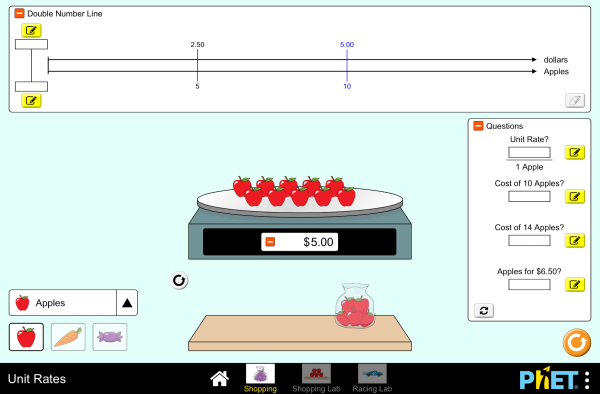# 单位价格下载嵌入代码 关闭 嵌入模拟程序控制 你可以通过改变嵌入的代码改变程序的宽度和高度 插入一张图片，当点击这个图片时启动仿真程序。

### 主题

• 比率
• 比例推理
• Double Number Line
• Unit Rate

### 描述

Discover the unit rate while shopping for fruits, vegetables, and candy. Construct a double number line and look for patterns. Challenge yourself on the race track as you compare cars with different rates!

### 示范 学习目标

• Interpret ratios of mixed units (e.g. \$/lb)
• Define the unit rate and determine a method to calculate it
• Predict how changing the numerator or denominator of a rate will affect the unit rate
• Use the double number line to reason about rates and solve real-world problems
• Develop strategies to use the unit rate to solve problems
• Compare unit rates between two simultaneous situations

### Standards Alignment

#### Common Core - Math

6.RP.A.2
Understand the concept of a unit rate a/b associated with a ratio a:b with b ≠ 0, and use rate language in the context of a ratio relationship. For example, "This recipe has a ratio of 3 cups of flour to 4 cups of sugar, so there is 3/4 cup of flour for each cup of sugar." "We paid \$75 for 15 hamburgers, which is a rate of \$5 per hamburger."1
6.RP.A.3
Use ratio and rate reasoning to solve real-world and mathematical problems, e.g., by reasoning about tables of equivalent ratios, tape diagrams, double number line diagrams, or equations.
6.RP.A.3b
Solve unit rate problems including those involving unit pricing and constant speed. For example, if it took 7 hours to mow 4 lawns, then at that rate, how many lawns could be mowed in 35 hours? At what rate were lawns being mowed?
6.RP.A.3d
Use ratio reasoning to convert measurement units; manipulate and transform units appropriately when multiplying or dividing quantities.

### 教师指南仿真程序控制概述,简化模型,学生视角的思考 ( PDF ).

### 教师提交的活动

Preguntas de razonamiento para todas las simulaciones HTML5Diana López K-5

Finding and Using Unit RatesKarina K. R. Hensberry 初中 指导 数学
Using Ratios and Rates to Solve ProblemsIan Whitacre, Sebnem Tekin 初中 指导 数学
Comparing Unit RatesIan Whitacre, Sebnem Tekin 初中 指导 数学
Grocery ShoppingDaniel Greenberg 初中 指导 数学
CCSS Math Alignment with SimsAmanda McGarry 初中

K-5

Mapping of PhET and IBDP Physics Jaya Ramchandani 高中 其它 物理学
Using Unit Rate to Understand Slope Karissa Gonzalez 初中

Finding Unit Rates Using Addition, Subtraction, Multiplication, and Division Bethany Konz 初中 指导 数学

### HTML5 程序：

iOS 8+ Safari

Android:

Chromebook:

Windows 系统:

Macintosh 系统:
OS 10.9.5 或之后的系统版本，Safari 9+版本，或者火狐浏览器，谷歌浏览器的最新版本均支持我们的程序。

Linux 系统:

### Java and Flash 程序：

Java 与 Flash程序可以在PC端，mac端，及linux系统上运行。具体的系统配置要求如下：

Windows 系统:
Windows 10, 8.1, 7

Macintosh 系统:
Mac OS X 10.9.5+

64位浏览器（例如Safari，火狐，谷歌等）

Linux 系统:
Oracle Linux 5.5+1
Oracle Linux 6.x (32位), 6.x (64位)2
Oracle Linux 7.x (64位)2
Red Hat Enterprise Linux 5.5+1, 6.x (32位), 6.x (64位)2
Ubuntu Linux 12.04 LTS, 13.x
Suse Linux Enterprise Server 10 SP2+, 11.x

• Amy Rouinfar (lead designer)
• Chris Malley (developer)
• Dave Schmitz (developer)
• Amy Hanson
• Amanda McGarry
• Susan Miller
• Ariel Paul
• Kathy Perkins
• almond-0.2.9.js
• base64-js-1.2.0.js
• easing-equations-r12
• FileSaver-b8054a2.js
• font-awesome-4.5.0
• game-up-camera-1.0.0.js
• jama-1.0.2
• jquery-2.1.0.js
• lodash-4.17.4.js
• pegjs-0.7.0.js
• seedrandom-2.4.2.js
• text-2.0.12.js
• TextEncoderLite-3c9f6f0.js
• Tween-r12.js